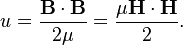Magnetic Force on Iron

I searched different places but couldn't find how actually you calculate this:-
What is the magnitude of force experienced by a cylindrical piece of iron placed near the end of a solenoid with internal field strength B0.

Staff Emeritus
2021 Award
The reason you keep asking this and not getting an answer is that the force is not dependent on the field strength. It's dependent on the gradient of the field strength. This is zero for an ideal (long) solenoid and complicated for a real one.

It's dependent on the gradient of the field strength.
Ok, then how do i calculate the force if I know the gradient of the field i.e. I know the function B(x,y,z).

Ok, then how do i calculate the force if I know the gradient of the field i.e. I know the function B(x,y,z).
One way to calculate the force is to examine how the total stored magnetic energy W of the system changes if the iron object is displaced by a distance dx. As you know, work (energy) is force F times distance dx. So the derivative of the stored energy W in the direction dx is the force in the direction dx:

Fx = dW/dx.

Smythe's (Static and Dynamic Electricity) exact solution for the axial field of a finite length solenoid is given in post #4 (see thumbnail) of this thread:

Bob S

Last edited:
Thanks for that. It really helped me.

One way to calculate the force is to examine how the total stored magnetic energy W of the system changes if the iron object is displaced by a distance dx. As you know, work (energy) is force F times distance dx. So the derivative of the stored energy W in the direction dx is the force in the direction dx:
Fx = dW/dx.
So can I proceed like this to calculate the force on a small iron piece lying some distance from the axis of the solenoid.?
1. The B inside the iron is multiplied by factor ur, So Biron=urBu0
2.The total Energy stored inside the piece can be calculated by intergrating over the entire volume of the iron piece
http://hyperphysics.phy-astr.gsu.edu/%E2%80%8Chbase/electric/imgele/engbf.gif [Broken]
3. When the iron piece is moved a small distance then then the total Energy Stored will change.

Then applying Fx = dW/dx. will give the force.

Last edited by a moderator:
I believe the magnetic energy density outside the iron is

(1/2μ0)∫B2 dV

and inside the iron it is

(1/2μμ0)∫B2 dV

so because B is continuous there is very little energy stored inside the iron.

But there is a dipole field outside the iron that I have not included.

I know there is a thread on this but I cannot find it.

Bob S

Inside the iron it is
(1/2μμ0)∫B2 dV
so because B is continuous there is very little energy stored inside the iron.
on the contrarary I think there is lot of energy inside the iron because
the total energy inside iron, as you pointed out, is
(1/2μμ0)∫B2 dV
But, B = μB0
So, the energy stored becomes
(1/2μμ0)∫(Bμ)2 dV
= (1/2μμ02∫B2 dV
= (μ/2μ0)∫B2 dV

When the piece of iron is moved a small distance towards the solenoid, the intensity of B increases, so does the energy stored inside the iron.
So, the iron shouldn't move towards the solenoid (since it creates increased energy).
This is directly against the fact that the iron piece is attracted towards the solenoid!!!??

Last edited:
on the contrarary I think there is lot of energy inside the iron because
the total energy inside iron, as you pointed out, is
(1/2μμ0)∫B2 dV
But, B = μB0
So, the energy stored becomes
(1/2μμ0)∫(Bμ)2 dV
= (1/2μμ02∫B2 dV
= (μ/2μ0)∫B2 dV

When the piece of iron is moved a small distance towards the solenoid, the intensity of B increases, so does the energy stored inside the iron.
So, the iron shouldn't move towards the solenoid (since it creates increased energy).
This is directly against the fact that the iron piece is attracted towards the solenoid!!!??
Err..... no. Very little energy is stored in the iron. Because the normal component of B is continuous, the energy density is higher in the air than in the iron. See thumbnail.
Bob S

Attachments

•Magnet_air_gap.jpg
38.8 KB · Views: 2,056
Last edited:
Err..... no. Very little energy is stored in the iron. Because the normal component of B is continuous, the energy density is higher in the air than in the iron.
Bob S
But In wikipaedia I read this:(here u is the total energy stored).
I am confused that, Is it B or H , that is same for both air core and iron-core solenoid?

The basic form for the energy stored in a magnetic field is

W = (1/2)∫ B H dV

In a magnetic system that has both iron and air in the same flux, only B (the normal component) is continuous at the air / iron interface. So we write

W = (1/2μrμ0)∫ B2 dV in iron, and

W = (1/2μ0)∫ B2 dV in air

Bob S